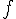# Hi/ho problem -- Lens magnification

• premed_love
In summary: Good luck with the rest of your studies!In summary, the conversation discussed the calculation of lateral magnification for an arrow placed perpendicular to the principal axis of a lens. The formula 1/f=1/di=1/do was used to find the image distance, di, and the magnification was calculated using the formula hi/ho=di/do. The discussion also mentioned the importance of considering the sign convention for the height of the image and being careful with significant figures in calculations.

## Homework Statement

An arrow h0 = 2.20 cm long is located 74.0 cm from a lens, which has a focal length= 30.6 cm. If the arrow is perpendicular to the principal axis of the lens, as shown in the figure below, what is its lateral magnification, defined as hi/h0?

I am given: ho=2.20 cm. do 74.0 cm. focal length is 30.6 cm

1/f=1/di=1/do

## The Attempt at a Solution

I used 1/f=1/di=1/do= 1/30.6=1/di+1/74.0 I get 52.175 with this equation which is my Di.
Then, I hi/2.20 cm=74.0/52.175 =3.12 from there I have to do hi/ho 3.12/2.20=1.418

I am completely wrong! Help![/B]

Not completely wrong. At least you got a number. (If you had ended up with an elephant, then yes, completely wrong.)

Your calculation for di looks good to me, although when you wrote your formula for the lens equation you have too many equal signs.

The calculation of the magnification is incorrect. Double-check your formula that relates hi and ho to di and do. You don't actually need to find the image height. You should be able to relate the magnification directly to the object and image distances.

Last edited:
I am still confused! Because if I relate it directly, then magnification is di/do therefore I get 1.418 which is still wrong

premed_love said:
I am still confused! Because if I relate it directly, then magnification is di/do therefore I get 1.418 which is still wrong
What value did you use for di? For do?

74.0/52.175

di goes on top. What was your value for di?

I was given do in the problem which was 74.0 cm, you may reread the problem to see if I am interpreting that correctly. I found di to be 52.175 which you said my math was right.

Yes, I believe your value for di (52.175 cm) is correct. The magnification is ##\frac{d_i}{d_o}##. So, what number goes on top?

TSny said:
Yes, I believe your value for di (52.175 cm) is correct. The magnification is ##\frac{d_i}{d_o}##. So, what number goes on top?

IN that case: it would be 52.175/74.0 = .70506 and that is wrong. Thank you for your help.

Are you supposed to include a - sign if the image is inverted?

I was struggling with the problem. I showed you my work. However, if you are also confused then I can not do anything about that. You tell me how to do di/do which I have done. I am not sure whether your supposed to show a sign.

The usual convention is to define the height of the image as negative if the image is inverted. Then the magnification formula is m = -di/ do. However, I did not know what convention you use in your class. In your first post you calculated an image height as positive and in post #3 you did not include a - sign. So, I wasn't sure of the sign convention you are using . Did you check your notes from class or your textbook? Anyway, hopefully the discussion has been beneficial. If not, please accept my apology.

TSny said:
The usual convention is to define the height of the image as negative if the image is inverted. Then the magnification formula is m = -di/ do. However, I did not know what convention you use in your class. In your first post you calculated an image height as positive and in post #3 you did not include a - sign. So, I wasn't sure of the sign convention you are using . Did you check your notes from class or your textbook? Anyway, hopefully the discussion has been beneficial. If not, please accept my apology.

Yes, I checked. I did my work correctly and used the negative sign three times! However, I was getting a wrong answer due to sig figs. That was frustrating because I never thought I had to be careful of sig figs!

Yes, that's frustrating. At least now you know to watch the sig figs.